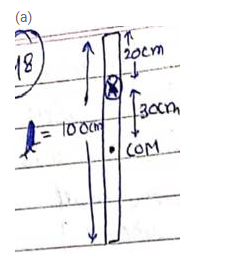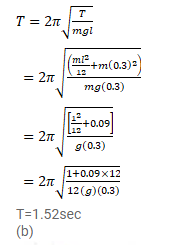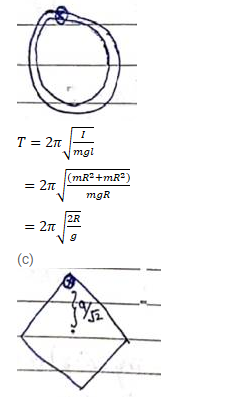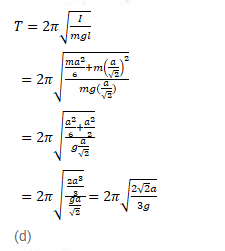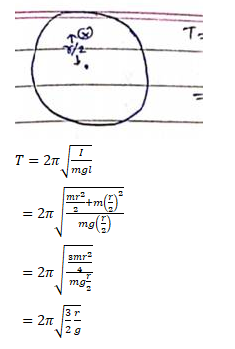# Find the time period of small oscillations of the following systems.

Question:

Find the time period of small oscillations of the following systems.

(a) A meter stick suspended through the $20 \mathrm{~cm}$ mark.

(b) A ring of mass $m$ and radius $r$ suspended through a point on its periphery.

(c) A uniform square plate of edge a suspended through a corner.

(d) A uniform disc of mass $m$ and radius $r$ suspended through a point $r / 2$ away from the center.

Solution: